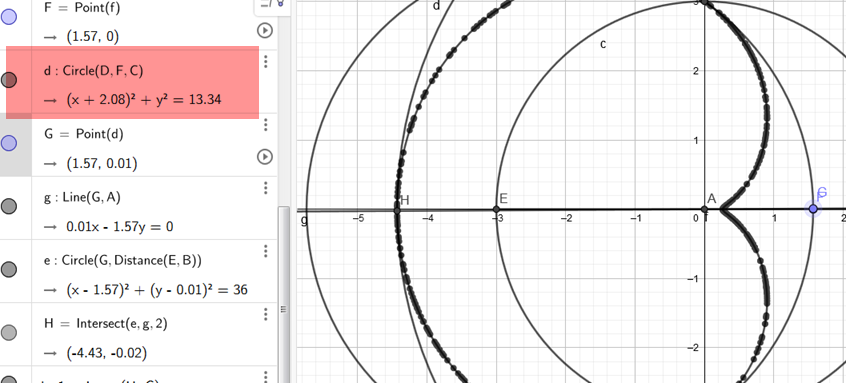# Trace a path into a curve

holyfssssd shared this question 2 years ago

I have the following setup:https://www.geogebra.org/cl...

As you move point G from D to C, point H will move along a path tracing a certain kind of curve.

Is there a way to convert this path into a proper curve parameter?1

it is a part of cardioid1

Thank you, what kind of equation did you use?1

Ah, I think I figured it out, it's Locus(H,G) right?1

Ok, the locus method works well in this case, but it becomes too laggy when there's a high quantity of them. Is there a way to use the cardioid equation? I tried to look up the equation, but I can't figure out how to fit the curve into my setup. Can anyone help me with this?1

What's the equation?1

The equation seems to be:

`(x^2+y^2-2ax)^2=4a^2.(x^2+y^2)`
Here's a few examples I found:

But there's also this one which uses

`Curve(cos(s) (1-cos(s)),sin(s) (1-cos(s)),s,0,t)`
https://www.geogebra.org/classic/dTw7UQEj

This site also lists other equations:

http://xahlee.info/SpecialP...1

This looks fine, try that inside Sequence()

`Curve(cos(s) (1-cos(s)),sin(s) (1-cos(s)),s,0,t)`1

How do I make the curve automatically match loc1 here? (even if you move point F):

https://www.geogebra.org/cl...